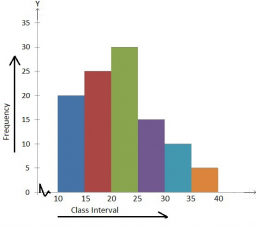# Students 34

Students were surveyed as part of a Statistics project to determine if younger adults are more likely to have tattoos. The results are listed in the two-way table below:

ageAt least one tattoo No tattoo Row totals
Age 18 - 29 165 342 507
Age 30 - 50 64 484 548
Column Totals 229 826 1055

What is the probability that a person is chosen at random:

a. ) Has at least one tattoo? =

b. ) Is between 30 - 50 years old? =

c. ) Is Age 18 - 29 AND has No tattoo =

d. ) Has at least one tattoo GIVEN they are between 30 - 50 years old? =

e. ) is between 18 - 29 years old, GIVEN they have at least one tattoo? =

f. ) Is between 18 - 29 years old OR has No tattoo? =

a =  229/1055
b =  548/1055
c =  342/1055
d =  64/229
e =  55/169
f =  991/1055

### Step-by-step explanation:Did you find an error or inaccuracy? Feel free to write us. Thank you!

Tips for related online calculators
Looking for help with calculating arithmetic mean?
Looking for a statistical calculator?
Need help calculating sum, simplifying, or multiplying fractions? Try our fraction calculator.
Would you like to compute the count of combinations?

#### Grade of the word problem:

We encourage you to watch this tutorial video on this math problem: